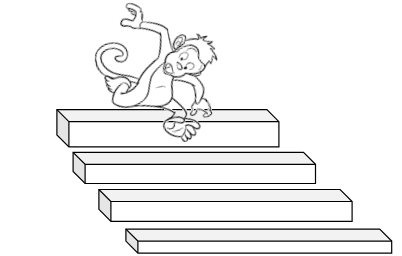# Ex.1.1 Q10 Integers - NCERT Maths Class 7

Go back to  'Ex.1.1'

## Question

A water tank has steps inside it. A monkey is sitting on the topmost step $$C$$ (i.e; the first step).The water level is on the ninth step.

i) He jumps $$3$$ steps down and then jumps back $$2$$ up. In how many jumps will he reach the water level?

ii) After drinking water he wants to go back. For this he jumps $$4$$ steps up and then jumps back $$2$$ steps down in every move. In how many jumps will he reach back the top step?

iii) If the number of steps moved down is represented by negative integers and the number of steps moved up by positive integers, represent his move in part(i) & (ii) by completing the following

$${\rm{a)\,\,-3 + 2 -}}...{\rm{ = -8}}$$

$${\rm{b)\,\,4-2 + \ldots }}{\rm{. = 8}}{\rm{.}}$$

In (a) the sum $$(-8)$$ represents going down by $$8$$ steps. So, what will the sum $$8$$ in (b) represent?Video Solution
Integers
Ex 1.1 | Question 10

## Text Solution

What is the known?

Number of steps going up and down.

What is unknown?

In how many jumps will he reach the water level and In how many jumps will he reach back the top step?

Steps:

i) Monkey jumps $$3$$ steps down and then back $$2$$ steps up. The jumps of monkey can be represented as:–

First jump $$=1+3+4 = 8$$ steps

Second jump $$=4–2 = 2$$ steps

Third jump $$=2+3 = 5$$ steps

Fourth jump $$=5–2 = 3$$ steps

Fifth jump $$=3+3 =6$$ steps

Sixth jump $$=6–2 =4$$ steps

Seventh jump $$=4+3 =7$$ steps

Eighth jump $$=7–2 =5$$ steps

Ninth jump $$=5+3 =8$$ steps

Tenth jump $$=8–2 =6$$ steps

Eleventh jump $$=6+3 =9$$ steps

Monkey will reach ninth step in $$11$$ jumps.

ii) Monkey jumps $$4$$ steps up and then jumps back $$2$$ steps down in every ove. The jumps of monkey can be represented as follows;–

First jump $$=9–4 =5$$ steps

Second jump $$=5+2 =7$$ steps

Third jump $$=7–4 =3$$ steps

Fourth jump $$=3+2 =5$$ steps

Fifth jump $$=5–4 =1$$ step

The monkey will reach back the top step after $$5$$ jumps.

iii) Moves in part (i)

\begin{align*} \left[\begin{array}{l} {-3+2-3+2-3} \\ {+2-3+2-3+2=-8} \end{array}\right] \end{align*}

which represents the monkey goes down by $$\rm{}8$$ steps

Moves in part (ii)

\begin{align}4–2+4–2+4=8,\end{align} the sum $$8$$ in (b) represents the monkey goes up by $$8$$ steps.

Learn from the best math teachers and top your exams

• Live one on one classroom and doubt clearing
• Practice worksheets in and after class for conceptual clarity
• Personalized curriculum to keep up with school